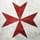It seems that you're using an outdated browser. Some things may not work as they should (or don't work at all).
We suggest you upgrade newer and better browser like: Chrome, Firefox, Internet Explorer or Opera

×
We’ve updated exchange rates and the current ones are as follows:

5.99\$ = 5.29 EUR = 4.49 GBP = 8.49 AUD = 459 RUB = 23.79 PLN = 54.7 SEK = 52.8 NOK = 39 DKK = 5.59 CHF = 7.69 CAD = 31.59 BRL = 38.00 CNY

9.99\$ = 8.79 EUR = 7.39 GBP = 14.09 AUD = 759 RUB = 39.69 PLN = 91.30 SEK = 88.00 NOK = 65.10 DKK = 9.29 CHF = 12.69 CAD = 52.59 BRL = 64.00 CNY

14.99\$ = 13.19 EUR = 11.09 GBP = 21.09 AUD = 1129 RUB = 59.49 PLN = 137.00 SEK = 132.10 NOK = 97.70 DKK = 13.89 CHF = 19.09 CAD = 79.00 BRL = 95.00 CNY
Has there been an unannounced price matrix update?

For non region-priced games I'm seeing

9.99\$ = 8.99EUR = 859RUB = 7.49GBP = 12.79CAD = 13.99AUD
We’ve updated exchange rates and the current ones are as follows:

5.99\$ = 5.39 EUR = 4.49 GBP = 8.39 AUD = 519 RUB = 24.89 PLN = 57.00 SEK = 53.8 NOK = 39.8 DKK = 5.59 CHF = 7.69 CAD = 30.39 BRL = 38.00 CNY

9.99\$ = 8.99 EUR = 7.49 GBP = 13.99 AUD = 859 RUB = 41.39 PLN = 95.10 SEK = 89.80 NOK = 66.30 DKK = 9.29 CHF = 12.79 CAD = 50.59 BRL = 63.00 CNY

14.99\$ = 13.39 EUR = 11.19 GBP = 20.95 AUD = 1279 RUB = 62.09 PLN = 142.70 SEK = 134.70 NOK = 99.50 DKK = 13.89 CHF = 19.23 CAD = 75.96 BRL = 95.00 CNY
We’ve updated exchange rates and the current ones are as follows:

5.99\$ = 5.49 EUR = 4.59 GBP = 8.19 AUD = 26.79 PLN = 59.00 SEK = 53.50 NOK = 40.70 DKK = 5.49 CHF = 7.69 CAD = 30.29 BRL = 38.00 CNY

9.99\$ = 9.19 EUR = 7.59 GBP = 13.59 AUD = 44.69 PLN = 98.30 SEK = 89.20 NOK = 67.90 DKK = 9.19 CHF = 12.79 CAD = 50.59 BRL = 63.00 CNY

14.99\$ = 13.79 EUR = 11.39 GBP = 20.39 AUD = 67.09 PLN = 147.60 SEK = 133.90 NOK = 101.90 DKK = 13.79 CHF = 19.09 CAD = 75.89 BRL = 95.00 CNY
Orpo (exclamation of surprise typical of the city of Gorizia, Italy). Beside the noticible disappearance of rubles, it looks quite bad for the Euro zone. How come another price raise after just on week? Honest question.
We’ve updated exchange rates and the current ones are as follows:

5.99\$ = 5,69 EUR = 4.79 GBP = 8.49 AUD = 25.79 PLN = 58.9 SEK = 57.4 NOK = 41.7 DKK = 5.79 CHF = 7.69 CAD = 28.89 BRL = 40.00 CNY

9.99\$ = 9,39 EUR = 7.99 GBP = 14.19 AUD = 43.09 PLN = 98.3 SEK = 95.8 NOK = 69.5 DKK = 9.69 CHF = 12.89 CAD = 48.19 BRL = 67.00 CNY

14.99\$ = 14,09 EUR = 11.99 GBP = 21.19 AUD = 64.59 PLN = 147.50 SEK = 143.8 NOK = 104.4 DKK = 14.49 CHF = 19.29 CAD = 72.39 BRL = 100.00 CNY
Not showing UK England currency.

Shows when i'm logged out but when i log in it goes to Euro.

I cleared my cookies and it didn't help.

edit:

nvm ,i missed some of the cookies during cookie removal and also forgot to go back into my locale settings and change to GBP.

GPD UK currency only appears in account settings once all cookies are cleared and you've logged in again.

Ok now.
Post edited June 24, 2022 by headholo
No info here, but price matrix has been probably updated.
I can see hundreds of games' prices increased in PLN by 8-9%...ciemnogrodzianin: No info here, but price matrix has been probably updated.
I can see hundreds of games' prices increased in PLN by 8-9%...
Yep.

\$5.99 = 5.89 EUR = 5.00 GBP = 8.79 AUD = 27.99 PLN = 61.60 SEK = 59.70 NOK = 5.89 CHF = 7.79 CAD = 32.49 BRL = 40.00 CNY

\$19.99 = 19.59 EUR = 16.69 GBP = 29.09 AUD = 93.39 PLN = 205.60 SEK = 199.40 NOK = 19.49 CHF = 25.89 CAD = 108.29 BRL = 135.00 CNY

Only have flat priced games of those two values written down, so that was what I could check.
But considering the rounding that applies for non-USD values, this near-parity also causes oddities at very low prices, for example EUR price being higher.
We’ve updated exchange rates and the current ones are as follows:

5.99\$ = 5.89 EUR = 5.00 GBP = 8.79 AUD = 27.99 PLN = 61.60 SEK = 59.70 NOK = 43.70 DKK = 5.89 CHF = 7.79 CAD = 32.49 BRL = 40.00 CNY

9.99\$ = 9.79 EUR = 8.39 GBP = 14.59 AUD = 46.69 PLN = 102.70 SEK = 99.60 NOK = 72.80 DKK = 9.79 CHF = 12.99 CAD = 54.19 BRL = 67.00 CNY

14.99\$ = 14.69 EUR = 12.49 GBP = 21.79 AUD = 69.99 PLN = 154.20 SEK = 149.50 NOK = 109.30 DKK = 14.59 CHF = 19.39 CAD = 81.29 BRL = 101.00 CNY